and ortutorials

# diffraction

Diffraction basics

Diffraction phenomenom is described by Huygens-Fresnel principle assuming that every point of a surface illuminated by a light wave can be considered as a secondary source emitting a spherical wave ( in all directions ). All these spherical waves interfere to generate the diffracted wave. A consequence of this principle is that a wave diffracted by an aperture has different phase and amplitude distribution than the incident wave, phase and amplitude distribution depending on the dimensions and shape of the aperture. The Fraunhofer diffraction is the particular case for which the wave is monochromatic and the aperture is plane. The Fraunhofer approximation is then used for the calculation of the diffracted wave and applies when the aperture and the diffracted pattern are small enough regarding their distance The simplest case is the diffraction of a plane wave observed at infinity.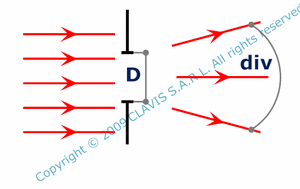The diffracted wave of a monochromatic plane wave by a spherical aperture perpendicular to the propagation axis is no more plane. It has a symmetry of revolution and a certain divergence. Its amplitude distribution is a Bessel function ( describing an Airy pattern ) of the observation angle while initial plane wave amplitude distribution is an Airy pic. Most energy is contained in the central bright zone of the pattern for angles smaller than i1, i1 corresponding the first zero of the Airy pattern. The diffracted wave divergence is assumed to be div = 2 i1. div depends on wavelength ( lam ) and aperture diameter ( D ).For a monochromatic plane wave diffracted by a rectangular slit, diffraction affects the wave only in the plane perpendicular to the slit. The amplitude of the diffracted wave is a "sinc" function of observation angle. Most energy is contained in the central bright band of the pattern corresponding to angles smaller than i1 ( i1 being the first zero of the "sinc" function ). The diffracted wave divergence is assumed to be div = 2 i1. div depends on the wavelength lam and the slit thickness a.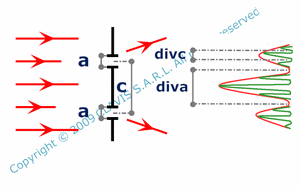Like in the precedent paragraph, for a monochromatic plane wave diffracted by two parallel and identical rectangular slits ( with a thickness a ) , diffraction affects the wave only in the plane perpendicular to the slits. Amplitude of the diffracted wave is the product of the interference pattern ( generated by two infinitely thin slits ) and the diffraction pattern ( generated by a lonely slit with the thickness a ). divc is the angular separation between two consecutive zero of the interference pattern and diva is the divergence of the wave diffracted by a lonely slit. diva and divc depend on the wavelength ( lam ), the slit thickness ( a ) and the distance between slit centers ( c ).For these three examples, it is possible to observe the diffraction pattern in the back focal plane of a lens. Depending on the aperture considered, the diffracted wave divergence is then transformed in diameter or width.

The central spot diameter Dd of a diffracted monochromatic plane wave by circular aperture ( diameter D ) observed in the back focal plane depends on D, lam but also fi, the effective focal length in the image space.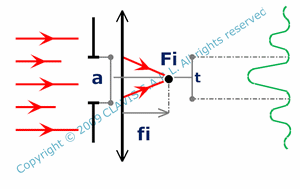When diffracted by a rectangular slit, the width of the brightest band ( t ) in the back focal plane depends on a, lam and fi.When diffracted by two parallel rectangular slits, the width Da of the brightest band of the diffracted pattern envelope and the pitch Dc of the interference pattern ( in the back focal plane ) depend on a, c, lam and fi.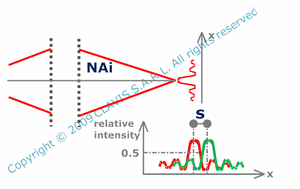An optical system has a limited size. Consequently, it has an aperture and diffracts light. Even if an optical system has no aberration, because of the diffraction, the image of a point is not a point but a spot. The image of a point can't be smaller than the diffraction spot. This limits the minimum size that can be resolved in the image plane ( power of resolution s ). It is possible to calculate s by using the Rayleigh criteria. s depends on the wavelength ( lam ) and the numerical aperture in the image space ( NAi ).

Diffraction by a gratingA grating is a periodic succession of parallel slits ( transmission grating ) or parallel reflecting thin bands ( reflective grating ). The pitch ( d ) is the distance between adjacent slits or reflective bands. Each slit or band acts as a diffractive element. Consequently, the wave diffracted by a grating is the result of intereferences between waves diffracted by each diffractive element.For an incident monochromatic plane wave, interefences are constructive only for light rays going in certain directions. Consequently, the diffracted wave can propagate only in these directions. Constructive interferences occur when the phase shift between waves coming from adjacents diffractive elements is 2kp where k is a positive or negative integer. k defines a propagation direction of the diffracted wave. k is the diffraction order. The diffraction angle id depends on the considered order ( k ) , the wavelength ( lam ), the angle of incidence ( i ) and the pitch ( d ). For a certain diffraction order k, a polychromatic plane wave is diffracted in as many directions as wavelengths. The wave spectrum is spatially dispersed by the grating. Then, a diffracted direction corresponds to a unique wavelength. However, there are some limitations. Because the diffraction order is not unique, the spectrum spatially dispersed by the grating in different diffraction orders may overlay. Considering a wavelength ( lam ), and a diffraction order ( k ), the free spectral range gives the maximum spectrum width without overlay with higher diffraction orders ( lam being the starting wavelength of the spectrum ).Because a grating has finite dimensions it acts as an aperture. Consequently, a plane wave diffracted in a certain order k is no more plane but has a certain divergence impacting the grating resolvance. The resolvance ( Re ) at a certain wavelength is the ratio of the wavelength on the smallest wavelength change that can be detected. It's a function of the pitch ( d ), the order ( k ) and the incident beam diameter ( D ).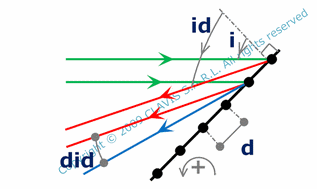The dispersion of a grating is also a useful parameter. Considering a plane incident monochromatic wave ( at the wavelength lam ), the angular dispersion ( did ) is the angle variation of the diffracted wave for a wavelength change of 1 nm. The angular dispersion ( did ) depends on the angle of incidence ( i ), the pitch ( d ), the wavelength ( lam ), the diffraction order ( k ) and and eventually the refraction index.Another useful parameter is the relation between incident and diffracted wave tilts. The diffracted wave tilt ( did ) is a function of the angle of incidence ( i ), the incident wave tilt ( di ), the diffraction order ( k ), the pitch ( d ), the wavelength ( lam ) and eventually the refraction index.

When the diffracted wave is observed through a lens, angles and angle shifts precedently discussed are transformed respectively in positions and distances depending also on the focal length of the considered lens.

References

"Optique Fondements et applications" - 2004 - author : José-Philippe Perez.

"Cours d'optique physique" - Institut d'optique théorique et appliquée - 1985 - author : Christian Imbert.

"Cours d'optique ondulatoire" - Université Denis Diderot Paris 7 - 2006 - author : G.Rebmann.

"Études graphiques des propriétés optiques des lames minces" - Journal de physique - 1950 - author : D. Malé.